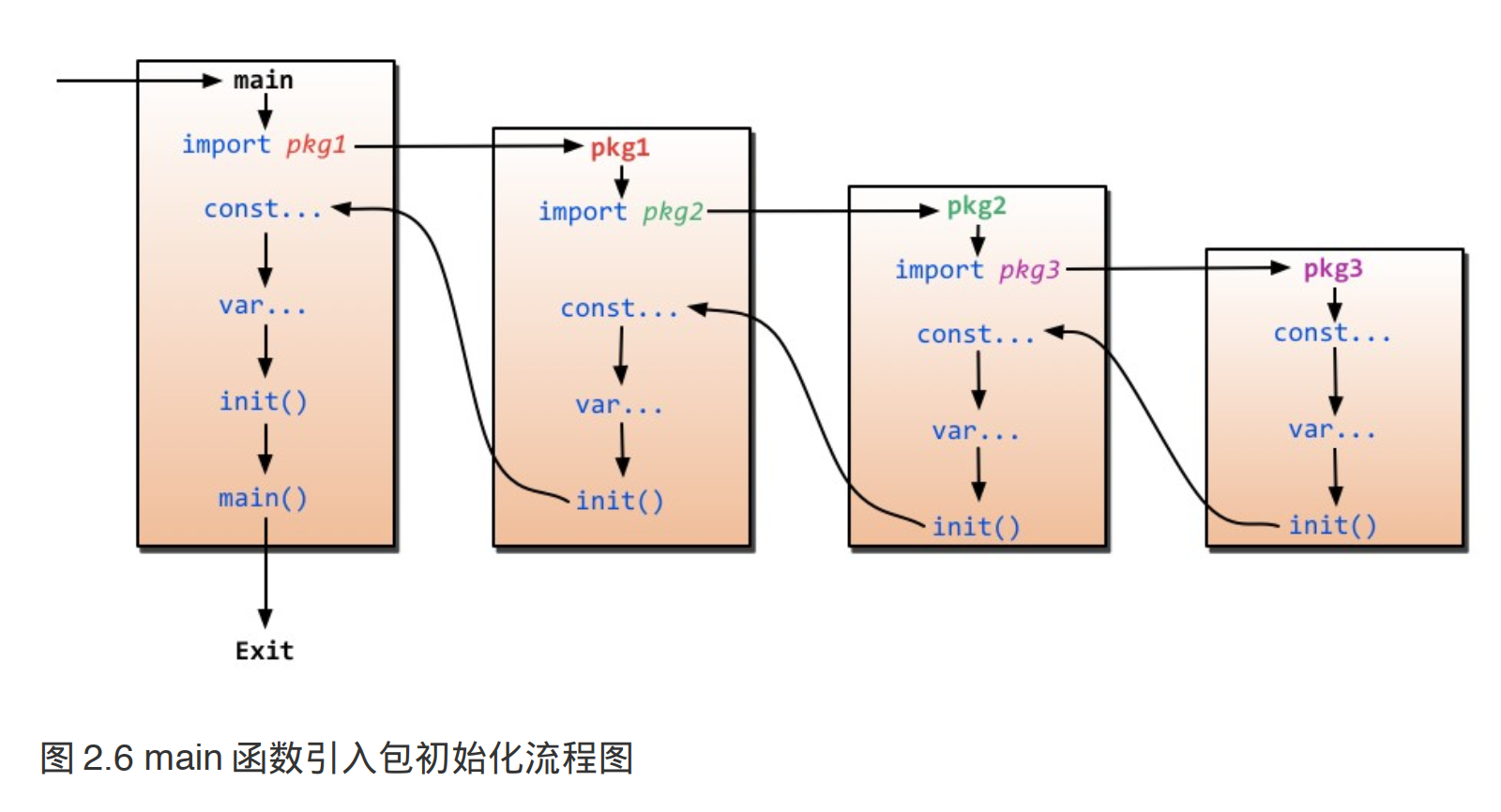7 Go语言函数

``````func hello(input1 type1, input2 type2) (output1 type1, output2 type2) {
//这里是处理逻辑代码
//返回多个值
return value1, value2
}``````

• 关键字 func 用来声明一个函数 `hello`

• 函数可以有一个或者多个参数，每个参数后面带有类型，通过`,`分隔

• 函数可以有多个返回值

• 上面返回值声明了两个变量 `output1``output2`，如果你不想声明也可以，直接就两个类型,比如 `func hello()(string,string){}`

• 如果只有一个返回值且不声明返回值变量，那么你可以省略 包括返回值 的括号,比如 `func hello() string { }`

• 如果没有返回值，那么就直接省略最后的返回信息，比如`func hello(){}`

• 如果有返回值， 那么必须在函数的外层添加 `return` 语句

``````package main

import "fmt"

func max(a int ,b int) int {
if a > b {
return a
}else {
return b
}
}

func main() {
x := 10
y := 20

maxValue := max(x,y)
fmt.Println("最大值为：",maxValue)
}
``````

### 多个返回值

Go 语言比 C 更先进的特性，其中一点就是函数能够返回多个值。 我们直接上代码看例子：

``````package main

import "fmt"

func maxAndMin(a int ,b int) (max int,min int) {
if a > b {
max = a
min = b
}else {
max = b
min = a
}

return max,min
}

func main() {
x := 10
y := 20

maxValue,minValue := maxAndMin(x,y)
fmt.Println("最大值为：",maxValue , " 最小值为：",minValue)
}
``````

``最大值为： 20  最小值为： 10``

### 变参

Go 函数支持变参。接受变参的函数是有着不定数量的参数的。为了做到这点，首先需要定义函数使其接受变参： `func myfunc(arg ...int) {}` `arg ...int` 告诉 Go 这个函数接受不定数量的参数。 注意，这些参数的类型全部是 `int`。 在函数体中，变量 `arg` 是一个 `int``slice`

``````for _, n := range arg {
fmt.Printf("And the number is: %d
", n)
}``````

### 传值与传指针

``````package main
import "fmt"
//简单的一个函数，实现了参数+1 的操作
a = a+1 // 我们改变了 a 的值
return a //返回一个新值
}

func main() {
x := 3
fmt.Println("x = ", x)     // 应该输出 "x = 3"
fmt.Println("x+1 = ", x1)  // 应该输出"x+1 = 4"
fmt.Println("x = ", x)     // 应该输出"x = 3"
}``````

``````package main
import "fmt"

//简单的一个函数，实现了参数+1 的操作
func add1(a *int) int { // 请注意，
*a = *a+1           // 修改了 a 的值
return *a           // 返回新值
}

func main() {
x := 3
fmt.Println("x = ", x)    // 应该输出 "x = 3"
fmt.Println("x+1 = ", x1)  // 应该输出 "x+1 = 4"
fmt.Println("x = ", x)     // 应该输出 "x = 4"
}``````

• 传指针使得多个函数能操作同一个对象。
• 传指针比较轻量级 `(8bytes)`,只是传内存地址，我们可以用指针传递体积大的结构 体。如果用参数值传递的话, 在每次 `copy`上面就会花费相对较多的系统开销（内存和时 间）。所以当你要传递大的结构体的时候，用指针是一个明智的选择。
• Go 语言中 `string``slice``map` 这三种类型的实现机制类似指针，所以可以直接传递，而不用取地址后传递指针。（注：若函数需改变 `slice` 的长度，则仍需要取地址传递指 针）

### defer

• Go 语言中有种不错的设计，即延迟（defer）语句，你可以在函数中添加多个 defer 语句。
• 当函数执行到最后时，这些 defer 语句会按照逆序执行，最后该函数返回。
• 特别是当你在进行一些打开资源的操作时，遇到错误需要提前返回，在返回前你需要关闭相应的资源，不然很容易造成资源泄露等问题。

``````func ReadWrite() bool {
file.Open("file")
// 做一些工作
if failureX {
file.Close()
return false
}

if failureY {
file.Close()
return false
}

file.Close()
return true
}
``````

``````func ReadWrite() bool {
file.Open("file")
defer file.Close()
if failureX {
return false
}
if failureY {
return false
}
return true
}``````

``````for i := 0; i < 5; i++ {
defer fmt.Printf("%d ", i)
}``````

### 函数作为值，类型

``````type typeName func(input1 inputType1 [, input2 inputType2 [, ...]) (result1 resultType1
[, ...])``````

``````package main
import "fmt"

type testInt func(int) bool // 声明了一个函数类型

//判断一个数是否是偶数
func isOdd(value int) bool {
if value % 2 == 0 {
return false
}
return true
}

//判断一个数是否是奇数
func isEven(value int) bool {
if value % 2 == 0 {
return true
}
return false
}

func filter(slice []int, f testInt) []int {
var result []int
for _, value := range slice {
if f(value) {
result = append(result, value)
}
}
return result
}

func main() {
slice := []int{1, 2, 3, 4, 5, 7}
fmt.Println("slice = ", slice)
odd := filter(slice, isOdd) // 函数当做值来传递了
fmt.Println("Odd elements of slice are: ", odd)
even := filter(slice, isEven) // 函数当做值来传递了
fmt.Println("Even elements of slice are: ", even)
}
``````

``````slice =  [1 2 3 4 5 7]
Odd elements of slice are:  [1 3 5 7]
Even elements of slice are:  [2 4]``````

### Panic 和 Recover

Go 没有像 Java 那样的异常机制，它不能抛出异常，而是使用了 `panic``recover` 机制。

#### Recover

``````var user = os.Getenv("USER")
func init() {
if user == "" {
panic("no value for \$USER")
}
}``````

``````func throwsPanic(f func()) (b bool) {
defer func() {
if x := recover(); x != nil {
b = true
}
}()
f() //执行函数 f，如果 f 中出现了 panic，那么就可以恢复回来
return
}``````

### main函数和 init函数

Go 里面有两个保留的函数：`init`函数和`main`函数

• init 函数（能够应用于所有的 package）

• main 函数（只能应用于 package main）。

• 这两个函数在定义时不能有任何的参数和返回值。

虽然一个 package里面可以写任意多个 init 函数，但这无论是对于可读性还是以后的可维护性来说，我们都强烈建议用户在一个 package 中每个文件只写一个 init 函数。

• Go 程序会自动调用 init()和 main()，所以你不需要在任何地方调用这两个函数。每个`package` 中的`init` 函数都是可选的,但 `package main` 就必须包含一个 `main` 函数。

• 程序的初始化和执行都起始于 main 包。
• 如果 `main` 包还导入了其它的包，那么就会在编译时 将它们依次导入。
• 有时一个包会被多个包同时导入，那么它只会被导入一次（例如很多包可 能都会用到 `fmt` 包，但它只会被导入一次，因为没有必要导入多次）。
• 当一个包被导入时，如果该包还导入了其它的包，那么会先将其它包导入进来
• 然后再对这些包中的包级常量和变量进行初始化
• 接着执行 `init` 函数（如果有的话），依次类推。
• 等所有被导入的包都加载完毕了，就会开始对 `main` 包中的包级常量和变量进行初始化
• 然后执行 `main` 包中的 `init`函数（如果存在的话），最后执行 `main` 函数。下图详细地解释了整个执行过程### import

``````import(
"fmt"
)``````

• 相对路径 `import "./model"` //当前文件同一目录的 model 目录，但是不建议这种方式来 import
• 绝对路径 `import "shorturl/model"` //加载gopath/src/shorturl/model 模块

#### `.` 操作，有时候我们会看到如下的方式导入包

``````import(
. "fmt"
)``````

#### 别名操作

``````import(
f "fmt"
)``````

#### `_` 操作

``````import (
"database/sql"
_ "github.com/ziutek/mymysql/godrv"
)``````Lv1

87

32

82